Rainfall Nowcast

# QPE (Quantitative Precipitation Estimates)

 Quantitative precipitation estimates (QPE) refers to the analysis of ground precipitation at locations or over a region of interest. SWIRLS provides several methods to compute QPE: Radar QPE Barnes analysis using rain gauge data Co-kriging analysis, with both rain gauge and radar data QPE is useful for generating rainfall distribution maps (also known as isohyet charts), which facilitates the general public and disaster mitigation agencies to appreciate the actual situation and estimate risks. QPE data may also be coupled with geophysical and hydrological models to predict and prevent landslides and floods.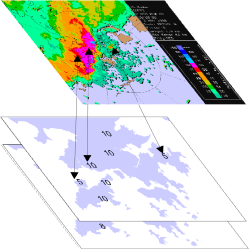Radar QPE is a real-time calibration approach for obtaining rainfall rate through the Z-R relation Z=aR^b. The coefficients a and b can be either static based on climatological values, or dynamically calibrated.
Barnes analysis interpolates data at grids from discrete rain gauge data with Gaussian weighting based on distance between data and estimation point: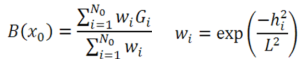Parameters Description
B Barnes estimation (mm)
N number of gauge report
G i-th gauge report
w weight of i-th gauge
h distance between gauge and estimation point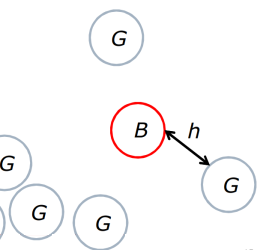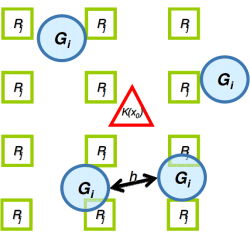Co-kriging analysis is a geostatistical technique to derive an optimal estimate of rainfall using both rain gauge and radar reflectivity data. While computationally intensive, co-kriging provides a sophisticated approach to estimate rainfall amount taking into account the error characteristics of rain gauge and radar measurements and their spatial correlation.

# Quality Control on Observations

 The weather radars and raingauges are maintained with regular calibration to ensure high data quality for input to the nowcasting systems. Automatic raingauge data are not only fundamental in quantitative rainfall analysis but also used as the ground truth in warning operation and forecast validation. Quality control is necessary before the data can be used quantitatively due to systematic and random errors. Substantial random errors and unreasonably small or false zero values can hamper accuracy of the analysis of precipitation and effective monitoring of heavy rain. To address the issue, SWIRLS incorporates a real-time rainfall data quality-control scheme based on co-kriging analysis when blending radar and raingauge data to generate the quantitative precipitation estimate (QPE). As a basis of the quality-control scheme, the co-kriging rainfall analysis was shown through a verification exercise to be superior to those obtained by the Barnes analysis and ordinary kriging of raingauge data. An example is illustrated below. Details of the algorithm are described in the paper Development of an operational rainfall data quality-control scheme based on radar-raingauge co-kriging analysisAn example of co-kriging rainfall analysis and raingauge data quality-control valid at 09:05 h on 7 June 2008 (top), along with the rainfall isohyet map based on the Barnes analysis method with only raingauge data (middle). A radar image at 09:00h (bottom) revealed significant rainfall in Victoria Harbour.

# QPF (Quantitative Precipitation Forecasts)

 Quantitative precipitation forecast (QPF) is the prediction of the amount of rainfall that a particular area is expected to receive. SWIRLS currently uses the advection-based model where QPF is calculated through the following steps: Compare two successive radar images and analyze the motion field with variational optical flow; Predict the evolution of radar echoes with semi-Lagrangian advection (SLA) of radar echoes; and Convert forecast radar reflectivity to rainfall intensity with static / dynamically calibrated Z-R relationship. Following calculation of forecast rainfall amount at grids, the predicted rainfall amounts at designated locations are interpolated. Products such as forecast rainfall map and time series of rainfall amount at selected location are then generated for forecasters' reference or dissemination to users.
 "Real-time Optical flow by Variational methods for Echoes of Radar (ROVER)" is a real-time variational optical flow scheme, which adopts 1) a pre-processing step to enhance radar reflectivity images; and 2) a real-time variational optical flow technique. 1.   Pre-processing step In ROVER, the reflectivity fields are enhanced by transforming the gridded value with the following function: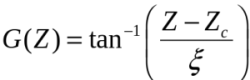where Zc and ξ control the point of inflection and its sharpness.Figure 1. Original (left) and Enhanced (right) reflectivity map 2.   Real-time variational optical flow technique To generate the motion field, SWIRLS adopts the open source codes titled "VarFlow" (Harmat, 2014), developed based on the algorithm proposed in Bruhn et al. (2003).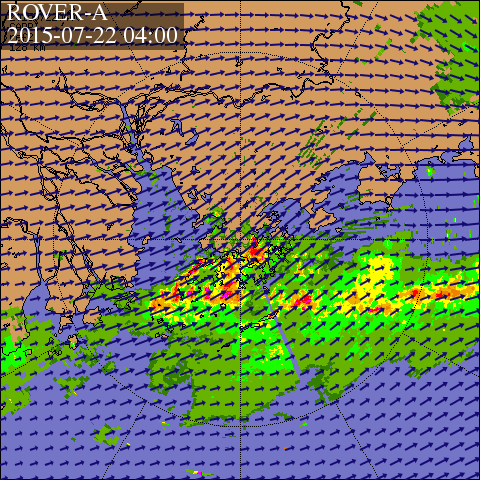Figure 2. Motion field with arrows indicating motion vectors
 After retrieval of the motion field, radar echoes are advected with backward semi-Lagrangian advection scheme.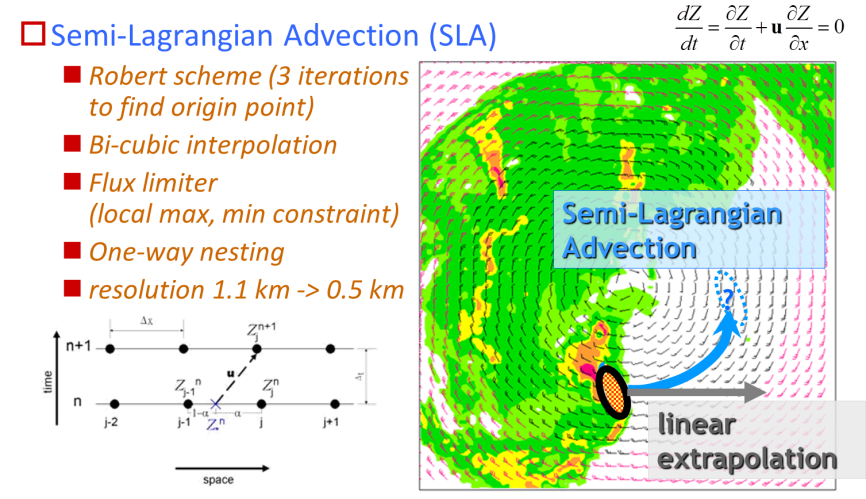Figure 3. Semi-Lagrangian Advection scheme adopted in SWIRLS
 Using Z-R relationship with static or dynamically calibrated parameters, radar reflectivity data are converted and aggregated to rainfall intensity data, such as hourly rainfall, which in turn are used by downstream applications to generate user products.
 Bruhn A, Weickert J, Feddern C, Kohlberger T, Schnörr C. 2003. Real-time optic flow computation with variational methods. In N. Petkov, M. A. Westenberg (Eds.): Computer Analysis of Images and Patterns. Lecture Notes in Computer Science, Vol. 2756, Springer, Berlin, 222-229. http://dx.doi.org/10.1007/978-3-540-45179-2_28 Harmat, A. 2010: Variational Optical Flow. http://sourceforge.net/projects/varflow/
 "Tracking Radar Echoes by Correlation (TREC)" essentially compares two successive radar reflectivity images and from which identifies a motion vector for each divided block of pixels through maximizing the correlation coefficient R, defined as follows: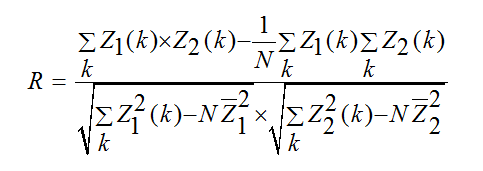where Z1 and Z2 are the array of pixels of reflectivity in the first field and in the second field respectively, and N denotes the number of data points within an array. In the implementation of SWIRLS, 93 × 93 overlapping blocks, each of size 19 × 19 pixels (i.e., N = 19 × 19) with the centers of two successive blocks separated by five pixels, are extracted from the 480 × 480 pixel reflectivity field, with each pixel representing an area of approximately 1.1 km × 1.1 km. For each block at time T, the correlation coefficients between itself and every other block within a searching radius of 19 pixels recorded 6 min earlier (i.e., T − 6 min) are computed. The block at time (T − 6 min) that yields the highest correlation coefficient with the block at time T is chosen, and the distance between the centers of these two blocks is then used to calculate the motion vector that represent the motion of the block at (T − 6 min). Repeating the procedure for each block, a motion field is obtained for all blocks with echoes. Cressman analysis is subsequently performed to assign motion vectors to those blocks without echoes.
 A radar echo tracking method utilizing variational optical flow technique, named as "Multi-scale Optical-flow by Variational Analysis (MOVA)", was developed in 2009. Defined as the apparent motion of brightness patterns in an image, optical flow would ideally equalize motion field barring lighting changes. Assuming that the brightness of a point of an object would remain unchanged along its path (i.e., "brightness constancy assumption"), the optical flow can be derived by solving the optical flow constraint (OFC) below: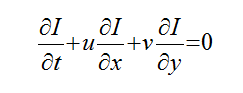where Z1 and Z2 are the array of pixels of reflectivity in the first field and in the second field respectively, and N denotes the number of data points within an array. In the implementation of SWIRLS, 93 × 93 overlapping blocks, each of size 19 × 19 pixels (i.e., N = 19 × 19) with the centers of two successive blocks separated by five pixels, are extracted from the 480 × 480 pixel reflectivity field, with each pixel representing an area of approximately 1.1 km × 1.1 km. For each block at time T, the correlation coefficients between itself and every other block within a searching radius of 19 pixels recorded 6 min earlier (i.e., T − 6 min) are computed. The block at time (T − 6 min) that yields the highest correlation coefficient with the block at time T is chosen, and the distance between the centers of these two blocks is then used to calculate the motion vector that represent the motion of the block at (T − 6 min). Repeating the procedure for each block, a motion field is obtained for all blocks with echoes. Cressman analysis is subsequently performed to assign motion vectors to those blocks without echoes. where I represents the brightness of a point projected on a two-dimensional plane. In MOVA, an algorithm named as the variational echo tracking (VET) developed by Germann and Zawadzki was adopted.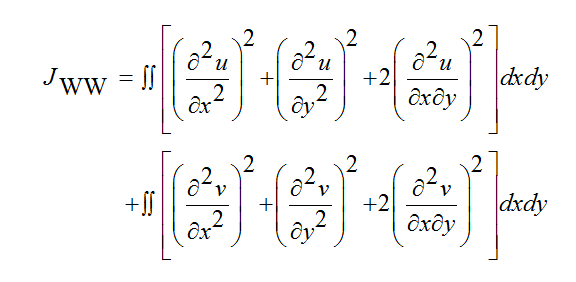There are seven cascading levels of various box sizes that range from 256 km down to 1.6 km are used together with the smoothness constraint. The smoothness constants for all level are controlled by seven parameters tuned for optimal performance, as given in the Table below: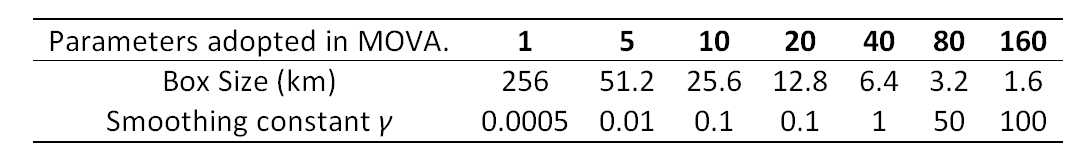When calculating the cost function, three consecutive radar reflectivity images are used to improve continuity of the retrieved motion field, i.e.,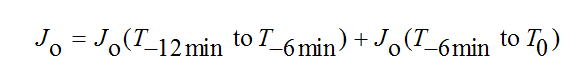While MOVA achieved improved overall performance over TREC, it has also been observed in operation that MOVA has a tendency to underestimate the speed of echo motion vectors, in particular at large scale, due to sub-optimal retrieval of echo motion in the minimization procedure. As a result, excessive rainfall was forecast at times. With a view to addressing the issue, another variational optical flow scheme, named as "Real-time Optical flow by Variational methods for Echoes of Radar (ROVER)", was used instead. In brief, ROVER is similar to MOVA but with two major enhancements: (1) having a pre-processing step to spatially smooth the radar reflectivity images; and (2) adoption of a variant type of optical flow technique. In convective systems, which dominate precipitation types in summer monsoon in Hong Kong, rain echoes could be so jumpy in reflectivity that their partial derivatives can hardly be accurately calculated. Stable estimates of the derivatives are only possible after the radar rain-rate fields are highlighted. In ROVER, the reflectivity fields are transformed with the following function: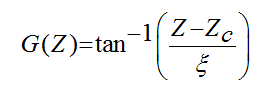where Zc and ξ control the point of inflection and its sharpness. In SWIRLS, Zc and ξ are empirically determined to be 33 dBZ and 4 dBZ, respectively based on a number of rainstorm cases in spring and summer. The transformation effectively enhances the contrast for reflectivity values in the range of 20–40 dBZ through suppressing the most and the least intense parts of rain bands. The other major enhancement is the adoption of the algorithm proposed in lieu of VET. An open source project named "VarFlow" provides the source codes used to implement the algorithm. The algorithm requires users to specify six parameters to control the Gaussian convolutions that spatially smooth radar images and motion fields to ensure tracking of the most critical features and to reduce jumpiness, to regularize smoothness constraints, to define the finest and coarsest levels, and to set the time intervals. The meanings of the parameters and the empirically determined parameters in ROVER are listed in the following Table.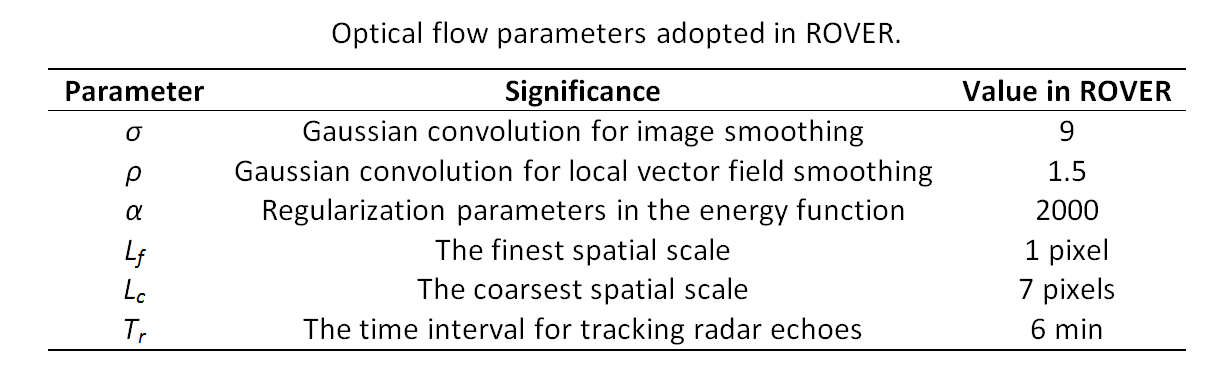As the choice of parameters would affect the quality of the motion field, the performance of the variational optical flow algorithm naturally depends on the parameters adopted. While it might be possible to derive a set of optimal parameters for a particular geographical setting, such a work would require a rich data archive and tremendous computational power to experiment with and explore all the dimensional space. It renders optimisation of parameters difficult if not impractical in practice. In our four years of operational experience, this set of parameters worked reasonably well in most of the time.

# QPF for Tropical Cyclone (TC) Rainfall

 To enhancing the performance of nowcast of rainfall brought by tropical cyclones, a new radar echo tracking scheme that separates the motion of the spiraling rain bands from the overall movement of tropical cyclone has been developed. Back-testing with historical cases in the past ten years reveals that the new scheme is more capable of preserving tropical cyclone rain band structures and can enhance forecast skills.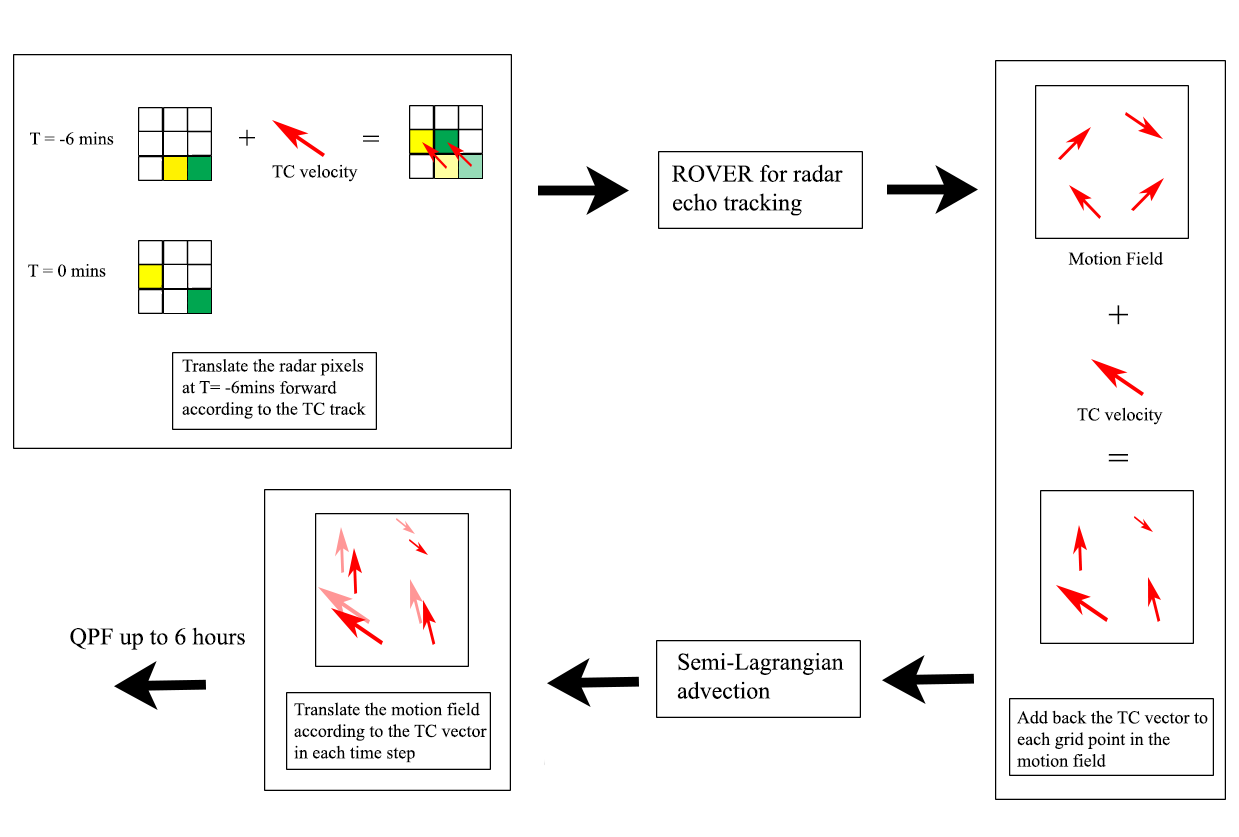An illustration on the workflow of the steps taken in the Enhanced Scheme Reference: W.C. Woo, K.K. Li, Michael Bala, 2014: An Algorithm to Enhance Nowcast of Rainfall Brought by Tropical Cyclones Through Separation of Motions. Tropical Cyclone Research and Review, 3(2), 111-121.